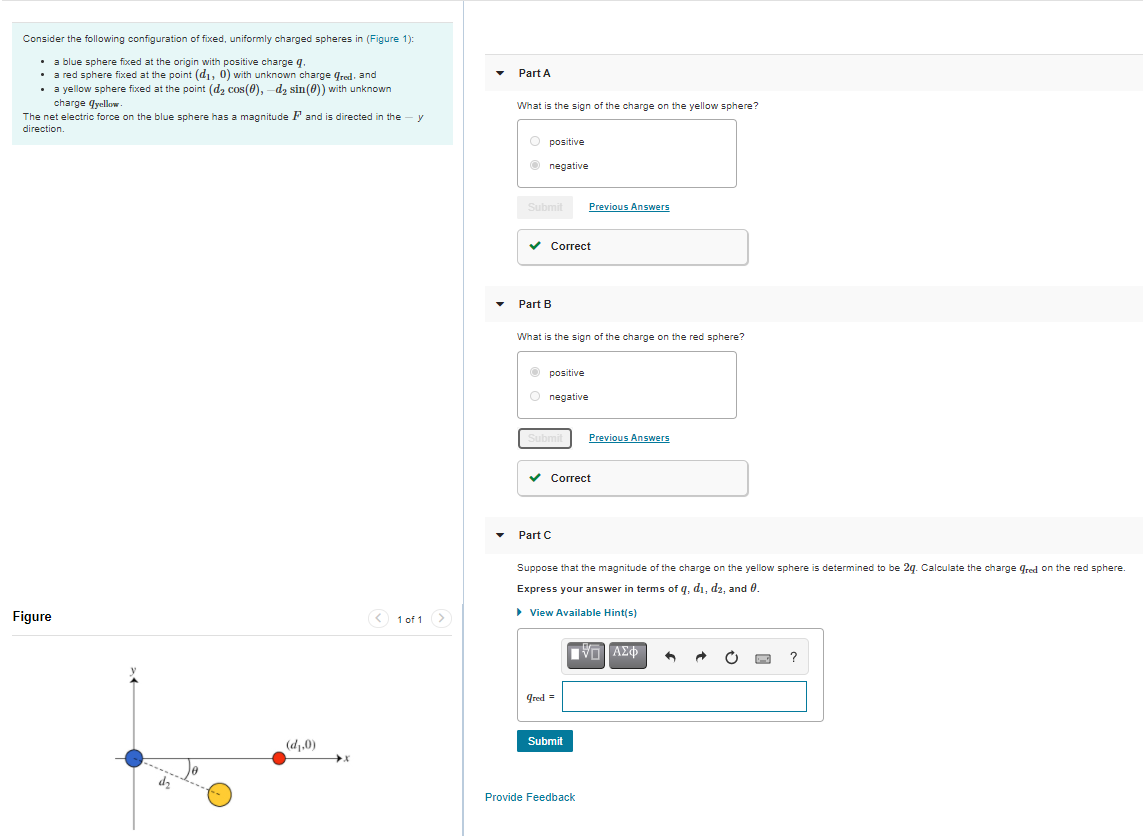# Question Solved1 AnswerConsider the following configuration of fixed, uniformly charged spheres in (Figure 1): Part A • a blue sphere fixed at the origin with positive charge q. • a red sphere fixed at the point (di, 0) with unknown charge fred, and • a yellow sphere fixed at the point (d, cos(Q), -d, sin(0)) with unknown charge yellow The net electric force on the blue sphere has a magnitude F and is directed in the - y direction What is the sign of the charge on the yellow sphere? positive negative Submit Previous Answers ✓ Correct Part B What is the sign of the charge on the red sphere? positive negative Previous Answers Correct Part C Suppose that the magnitude of the charge on the yellow sphere is determined to be 29. Calculate the charge Gred on the red sphere Express your answer terms of q, di, d2, and 0. Figure < 1 of 1 > View Available Hints) IVO AEO ? Cred = (d,.0) Submit Provide FeedbackTranscribed Image Text: Consider the following configuration of fixed, uniformly charged spheres in (Figure 1): Part A • a blue sphere fixed at the origin with positive charge q. • a red sphere fixed at the point (di, 0) with unknown charge fred, and • a yellow sphere fixed at the point (d, cos(Q), -d, sin(0)) with unknown charge yellow The net electric force on the blue sphere has a magnitude F and is directed in the - y direction What is the sign of the charge on the yellow sphere? positive negative Submit Previous Answers ✓ Correct Part B What is the sign of the charge on the red sphere? positive negative Previous Answers Correct Part C Suppose that the magnitude of the charge on the yellow sphere is determined to be 29. Calculate the charge Gred on the red sphere Express your answer terms of q, di, d2, and 0. Figure < 1 of 1 > View Available Hints) IVO AEO ? Cred = (d,.0) Submit Provide Feedback
More
Transcribed Image Text: Consider the following configuration of fixed, uniformly charged spheres in (Figure 1): Part A • a blue sphere fixed at the origin with positive charge q. • a red sphere fixed at the point (di, 0) with unknown charge fred, and • a yellow sphere fixed at the point (d, cos(Q), -d, sin(0)) with unknown charge yellow The net electric force on the blue sphere has a magnitude F and is directed in the - y direction What is the sign of the charge on the yellow sphere? positive negative Submit Previous Answers ✓ Correct Part B What is the sign of the charge on the red sphere? positive negative Previous Answers Correct Part C Suppose that the magnitude of the charge on the yellow sphere is determined to be 29. Calculate the charge Gred on the red sphere Express your answer terms of q, di, d2, and 0. Figure < 1 of 1 > View Available Hints) IVO AEO ? Cred = (d,.0) Submit Provide Feedback